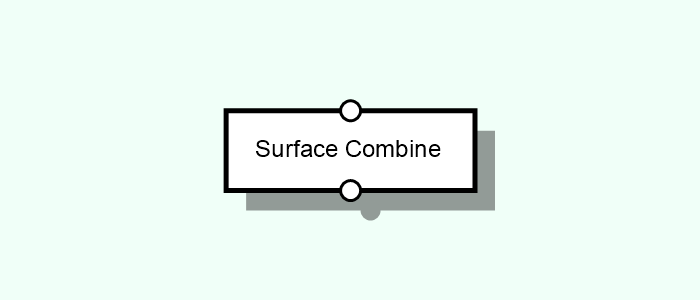# Surface Combine

Performs data combination - addition, subtraction and set - between two overlapping surfaces.Tags: Surface

## Parameters#

Input [Select]
The type of input port.
Setting a Single (square) input means that the node will be executed once for all surfaces. Useful to combine any collection of surfaces at once.
Setting a Dual (circles) input means that the node will be executed once for each pair of surfaces. Useful to combine exactly two surfaces at once.
Dual [Compound] [Adds ports 1, 2]
Processes two Surface entities at a time.
Processes all Surface entities at a time.
Operation [Choice]
The surface operation to perform:
Add means that the values from the surface A will be added to the ones from surface B for the overlapping areas.
Set means that the values from the surface A will be set to the ones from surface B for the overlapping areas.
Subtract means that the values from the surface B will be subtracted from the ones at surface A (i.e. the result is A - B).
Average means that the values from the surface A will be averaged with the ones from surface B for the overlapping areas.
Result [Choice]
Determines what the resulting surface should be:
First means that the result will have the position and dimensions of the first surface and the data operations will apply to this area only.
Intersection means that the result will have the position and dimensions of the area corresponding to the intersections between all surfaces. The data operations will apply to this area only.
Union means that the result will have the position and dimensions of the whole bounding area. The data operations will apply to the whole area, meaning that some data may be set to the default (such as zero values).

## Inputs#

This node has no native inputs.

### Parameter Inputs#

•  First [Single | Surface]: Processes one Surface entity at a time.
•  Second [Single | Surface]: Processes one Surface entity at a time.
•  Collective [Collective | Surface]: Processes all Surface entities at a time.

## Outputs#

• Output [Surface]: A new surfaces whose size encompasses the bounding area of all the input surfaces.